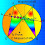## Saturday, September 30, 2017

### Geometry Problem 1348: Three Concentric Circles, Equilateral Triangle, Perpendicular Bisector, Congruence

Geometry Problem. Post your solution in the comment box below.
Level: Mathematics Education, High School, Honors Geometry, College.

Details: Click on the figure below.1.Drop perpendiculars from O to EA & B to DA
Since BA=OA => m(BAD) = m(OAE)
Hence triangles BAD & OAE are congruent
=> DA = EA
m(DAE)=m(DAO)+m(OAE)
=>m(DAE)=60
Hence DAE is equilateral

1.2.It is just construction of an equilateral triangle with vertices onto 3 concentric circles

2.Let a circle (A,AD) that intersects the circle C_3 at the point Z.Then triangle DBA=triangle ZOA (AD=AZ,AB=AO,DB=ZO).So triangle DAZ is
equilateral.If ZN perpendicular of AD,then AN=ND or N,M=coincide.Therefore and
the points Z,E= coincide.So triangle DAE is equilateral .
APOSTOLIS MANOLOUDIS 4 HIGH SCHOOL OF KORYDALLOS PIRAEUS GREECE